Question

# Two parallel plates, each having area A 3676cm are connected to the terminals of a battery of voltage V, 6 V A as shown...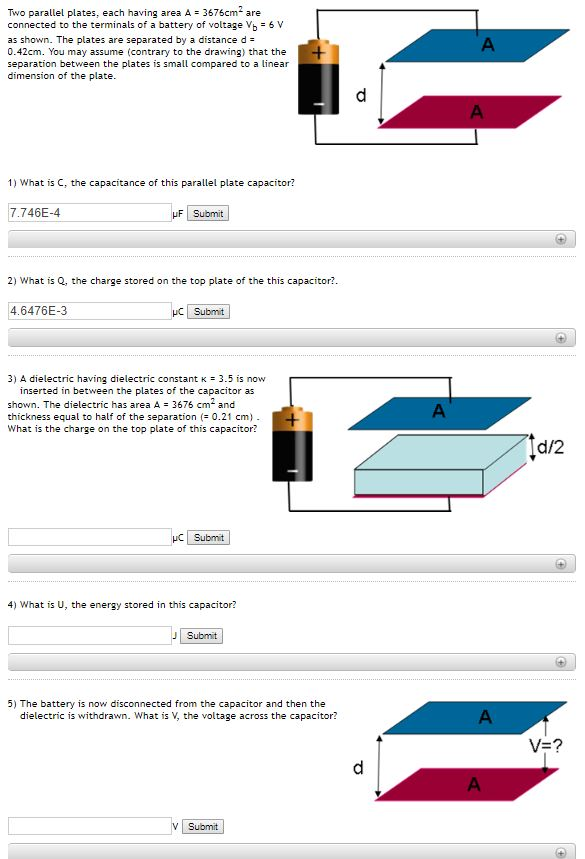Two parallel plates, each having area A 3676cm are connected to the terminals of a battery of voltage V, 6 V A as shown. The plates are separated by a distance d 0.42cm. You may assume (contrary to the drawing) that the separation between the plates is small compared to a linear dimension of the plate + A 1) What is C, the capacitance of this parallel plate capacitor? 7.746E-4 uF Submit 2) What is Q, the charge stored on the top plate of the this capacitor? uC Submit 4.6476E-3 3) A dielectric having dielectric constant K 3.5 is now inserted in between the plates of the capacitor as shown. The dielectric has area A 3676 cm and thickness equal to half of the separation (0.21 cm) What is the charge on the top plate of this capacitor? A Id/2 uc Submit 4) What is U, the energy stored in this capacitor? Submit 5) The battery is now disconnected from the capacitor and then the A dielectric is withdrawn. What is V, the voltage across the capacitor? V? A VSubmit TO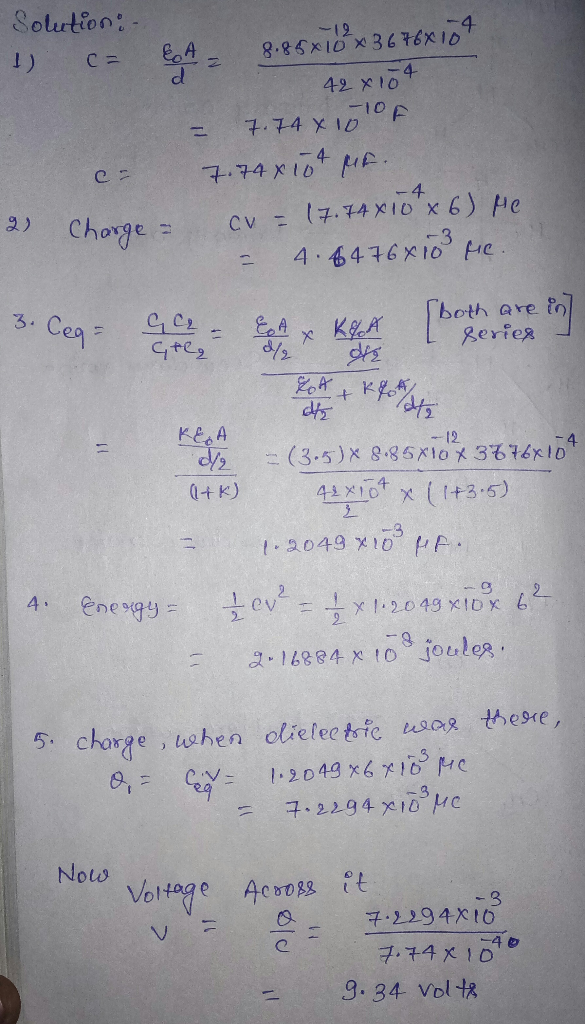Thank you for this man you are a legend

#### Earn Coins

Coins can be redeemed for fabulous gifts.

Similar Homework Help Questions
• ### Two parallel plates, each having area A- 2737cm are connected to the terminals of a battery...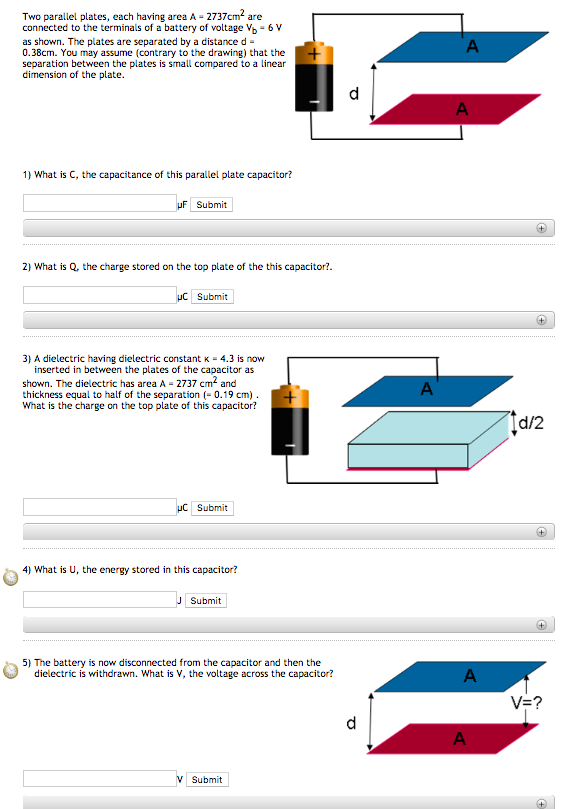Two parallel plates, each having area A- 2737cm are connected to the terminals of a battery of voltage Vb 6 V as shown. The plates are separated by a distance d 0.38cm. You may assume (contrary to the drawing) that the separation between the plates is small compared to a linear dimension of the plate 1) What is C, the capacitance of this parallel plate capacitor? Submit 2) What is Q, the charge stored on the top plate of the...

• ### Two parallel plates, each having area A = 2082cm2 are connected to the terminals of a...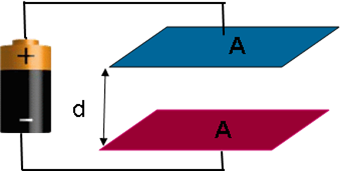Two parallel plates, each having area A = 2082cm2 are connected to the terminals of a battery of voltage Vb = 6 V as shown. The plates are separated by a distance d = 0.54cm. You may assume (contrary to the drawing) that the separation between the plates is small compared to a linear dimension of the plate. 1)What is C, the capacitance of this parallel plate capacitor?μF 2)What is Q, the charge stored on the top plate of the...

• ### Two parallel plates, each having area A 2625 em are connected to the terminals of a...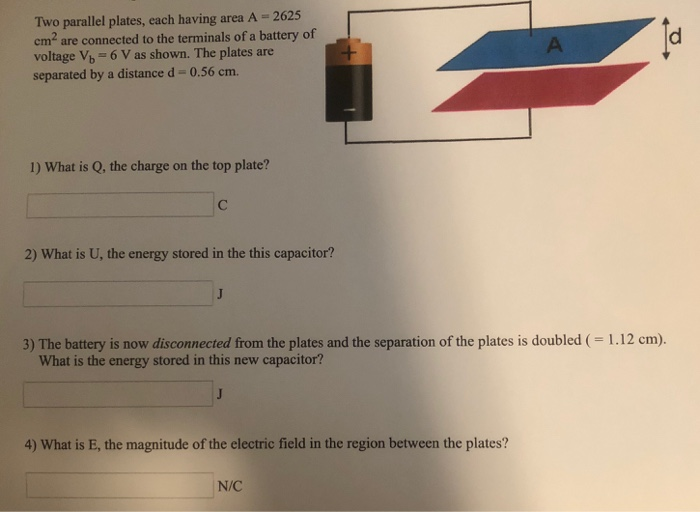Two parallel plates, each having area A 2625 em are connected to the terminals of a battery of voltage V6 6 V as shown. The plates are separated by a distance d-0.56 cm. 1) What is Q, the charge on the top plate? 2) What is U, the energy stored in the this capacitor? 3) The battery is now disconnected from the plates and the separation of the plates is doubled ( 1.12 cm). What is the energy stored in...

• ### Two parallel plates, each having area A = 3492 cm2 are connected to the terminals of...

Two parallel plates, each having area A = 3492 cm2 are connected to the terminals of a battery of voltage Vb = 6 V as shown. The plates are separated by a distance d = 0.52 cm. The battery is now disconnected from the plates and the separation of the plates is doubled ( = 1.04 cm). What is the energy stored in this new capacitor? (<= I know the answer to the previous question, but am having a hard...

• ### 6. A parallel-plate capacitor has plates of area 500 cm2 and is connected across the terminals...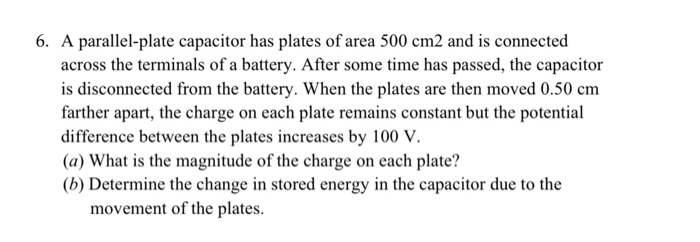6. A parallel-plate capacitor has plates of area 500 cm2 and is connected across the terminals of a battery. After some time has passed, the capacitor is disconnected from the battery. When the plates are then moved 0.50 cm farther apart, the charge on each plate remains constant but the potential difference between the plates increases by 100 V. (a) What is the magnitude of the charge on each plate? (b) Determine the change in stored energy in the capacitor...

• ### A parallel-plate capacitor has plates of area 400 cm2 and is connected across the terminals of...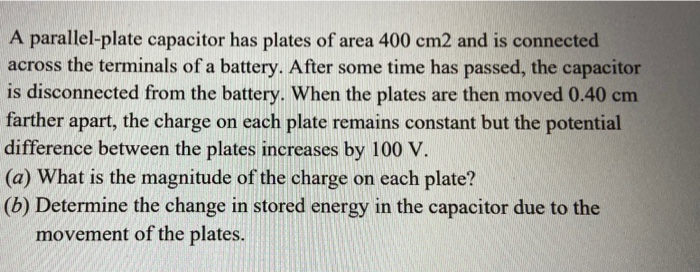A parallel-plate capacitor has plates of area 400 cm2 and is connected across the terminals of a battery. After some time has passed, the capacitor is disconnected from the battery. When the plates are then moved 0.40 cm farther apart, the charge on each plate remains constant but the potential difference between the plates increases by 100 V. (a) What is the magnitude of the charge on each plate? (5) Determine the change in stored energy in the capacitor due...

• ### Ez12/2 zbom / 3.3 A pair of parallel plates are connected to a 12-V battery and...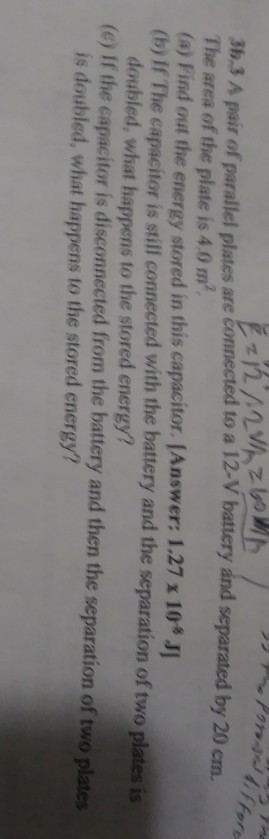Ez12/2 zbom / 3.3 A pair of parallel plates are connected to a 12-V battery and separated by 20 cm The area of the plate is 4,0 m, () Find out the energy stored in this capacitor. Answer: 1.27 x 10JI (b) If The capacitor is still connected with the battery and the separation of two plates is doubled, what happens to the stored energy? (ey if the capacitor is disconnected from the battery and then the separation of two...

• ### An air-filled parallel-plate capacitor has plate area A andplate separation d. The capacitor is connected...

An air-filled parallel-plate capacitor has plate area A and plate separation d. The capacitor is connected to a battery that creates a constant voltage V.A) Find the energy U_0 stored in the capacitor. Express your answer in terms of A, d, V, and ϵ_0.B) The capacitor is now disconnected from the battery, and the plates of the capacitor are then slowly pulled apart until the separation reaches 3d. Find the new energy U_1 of the capacitor after this process. Express...

• ### A parallel-plate capacitor with plate area 4.60 cm2 and air-gap separation 0.78 mm is connected to...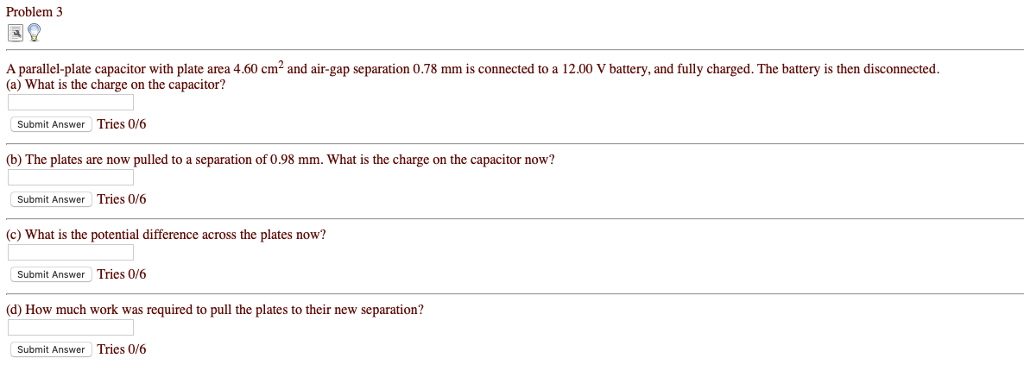A parallel-plate capacitor with plate area 4.60 cm2 and air-gap separation 0.78 mm is connected to a 12.00 V battery, and fully charged. The battery is then disconnected. (a) What is the charge on the capacitor? (b) The plates are now pulled to a separation of 0.98 mm. What is the charge on the capacitor now? (c) What is the potential difference across the plates now? (d) How much work was required to pull the plates to their new separation?...

• ### 9. An empty parallel plate capacitor is connected between the terminals of a 12.1-V battery and...

9. An empty parallel plate capacitor is connected between the terminals of a 12.1-V battery and charged up. The capacitor is then disconnected from the battery, and the spacing between the capacitor plates is quadrupled. As a result of this change, what is the new voltage between the plates of the capacitor? ----------- V